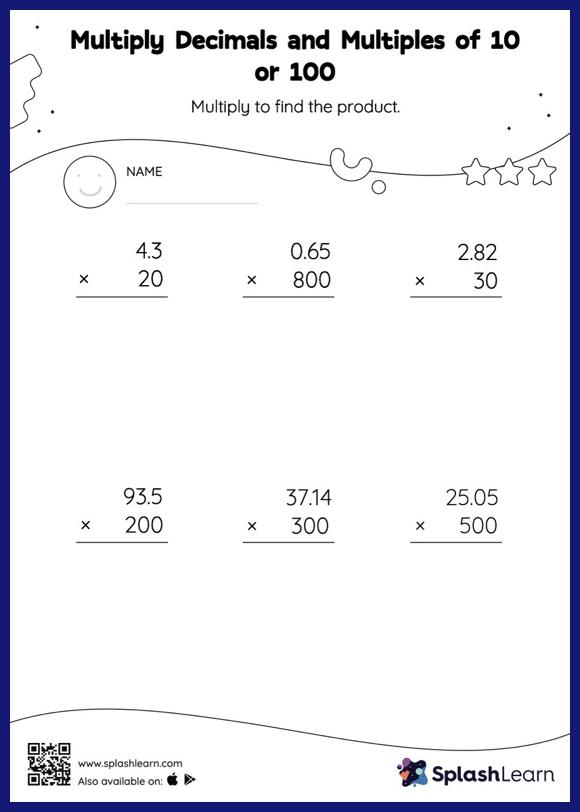# Multiply Decimals and Multiples of 10 or 100: Vertical Multiplication Worksheet

Home > Multiply Decimals and Multiples of 10 or 100: Vertical MultiplicationThis worksheet challenges young mathematicians to hone their math skills by solving a set of problems on multiplying decimals and multiples of 10 or 100. When students multiply a decimal by a number ending in zeros, they first ignore the decimal point, multiply the numbers, and then add the decimal point in the product as many places from the right as the number of decimal digits in the original number.